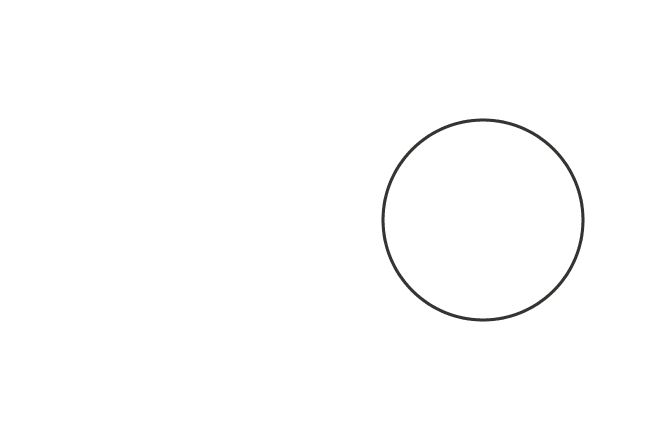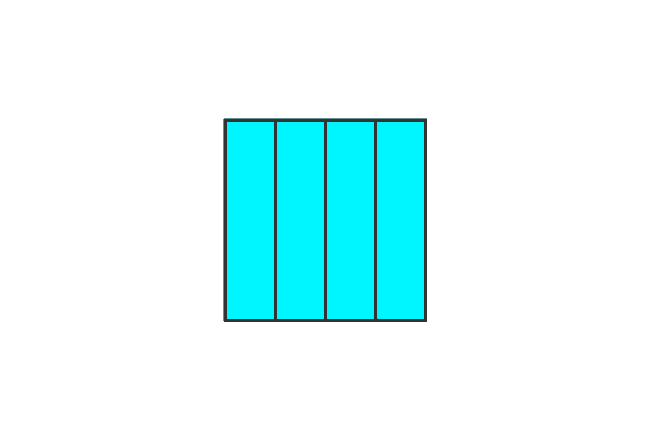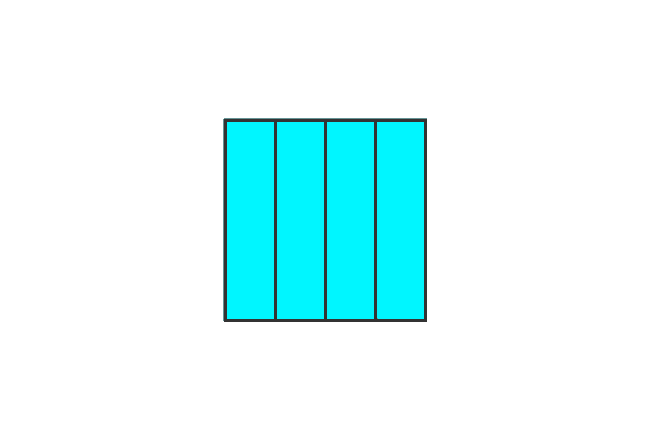# Improper fraction

A fraction whose numerator is greater than or equal to its denominator is called an improper fraction.

## Introduction

The meaning of improper fraction is defined from the meanings of the words “Improper” and “Fraction”.

1. The “Improper” means unacceptable, inappropriate, and so on.
2. The “Fraction” means a part of the whole quantity.

As per the meanings of them, it is not acceptable to consider either a whole quantity or the sum of a whole quantity and a part of another whole quantity as a fraction. So, the unacceptable fraction is called as an improper fraction.

### Formula

According to the theory of the improper fraction.

The quantity of an improper fraction is called by comparing its quantity of total number of selected parts with the number of parts of a whole quantity. It’s actually done by the ratio in mathematics.

$Number \, of \, Selected \, parts$ $\,\geq\,$ $Total \, number \, of \, Parts \, of \, a \, Quantity$

$\implies$ $\dfrac{Number \, of \, Selected \, parts}{Total \, number \, of \, Parts \, of \, a \, Quantity}$ $\,\geq\,$ $1$

$\,\,\, \therefore \,\,\,\,\,\,$ $Improper \, fraction$ $\,=\,$ $\dfrac{Number \, of \, Selected \, parts}{Total \, number \, of \, Parts \, of \, a \, Quantity}$ $\,\geq\,$ $1$

### Examples

An improper fraction is completely opposite to the proper fraction. The following examples help you to understand how fractions are formed improperly.

#### Example – 1

Consider a circle and split it as three equal parts. Now, let’s calculate the improper fraction mathematically.The total number of parts of a circle is $3$, take all three parts and then evaluate the fraction.

Fraction $\,=\, \dfrac{3}{3}$

$\implies$ Fraction $\,=\, \require{cancel} \dfrac{\cancel{3}}{\cancel{3}}$

$\,\,\, \therefore \,\,\,\,\,\,$ Fraction $\,=\, 1$

It is calculated that the fraction $\dfrac{3}{3}$ is equal to $1$ but the number $1$ is an integer and it represents a whole quantity.

So, it is not proper to consider $\dfrac{3}{3}$ as a fraction. Therefore, the fraction $\dfrac{3}{3}$ or $1$ is called an improper fraction. It is proved that any positive integer or a whole number is called as an improper fraction.

#### Whole Quantity & One partConsider two squares. Take, all parts from one square and a part from another square.

Fraction = $\dfrac{4}{4} + \dfrac{1}{4}$ = $\dfrac{5}{4}$

It is written as $5 : 4$ in ratio form. In this case, the value of numerator is greater than its denominator ($5 > 4$). Therefore, it’s known as an improper fraction.

Improper fraction = $\dfrac{5}{4}$

#### Whole Quantity & Two partsTake, all parts from one square and two parts from another square.

Fraction = $\dfrac{4}{4} + \dfrac{2}{4}$ = $\dfrac{6}{4}$

It’s written as $6 : 4$ in ratio form. The value in the numerator is greater than its denominator ($6 > 4$). So, it’s considered as an improper fraction.

Improper fraction = $\dfrac{6}{4}$

#### Whole Quantity & Three partsConsider, all parts from one square and three parts from second square.

Fraction = $\dfrac{4}{4} + \dfrac{3}{4}$ = $\dfrac{7}{4}$

It’s expressed as $7 : 4$ in ratio form mathematically. In this example, the value in the numerator is greater than its denominator ($7 > 4$). Hence, it’s known as an improper fraction.

Improper fraction = $\dfrac{7}{4}$

#### Whole QuantitiesNow, take all parts from two whole quantities.

Fraction = $\dfrac{4}{4} + \dfrac{4}{4}$ = $\dfrac{8}{4}$

It can be written in ratio form as $8 : 4$ in mathematics. The value in the numerator is greater than its denominator ($8 > 4$) and the fraction is known as an improper fraction.

Improper fraction = $\dfrac{8}{4}$

Mathematically, the quantity of this fraction is $2$, which is a whole number but it’s considered as a fraction whenever a whole quantity is written in the form a rational number.

Therefore, the quantities $\dfrac{4}{4}$, $\dfrac{5}{4}$, $\dfrac{6}{4}$, $\dfrac{7}{4}$ and $\dfrac{8}{4}$ are improperly called as fractions and they’re also called as improper fractions due to either same or greater value in the numerator when it’s compared with the value in the denominator.

It’s cleared that the value in the numerator is always greater than or equal to its denominator in the case of an improper fraction. Similarly, any whole number which is expressed in as a rational number, is considered as an improper fraction as well.

Latest Math Topics
Jun 26, 2023
Jun 23, 2023

Latest Math Problems
Jul 01, 2023
Jun 25, 2023
###### Math Questions

The math problems with solutions to learn how to solve a problem.

Learn solutions

Practice now

###### Math Videos

The math videos tutorials with visual graphics to learn every concept.

Watch now

###### Subscribe us

Get the latest math updates from the Math Doubts by subscribing us.